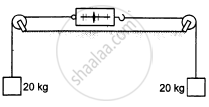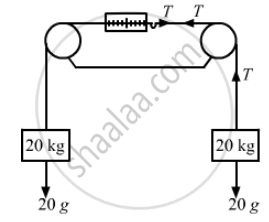# The Figure Shows a Light Spring Balance Connected to Two Blocks of Mass 20 Kg Each. the Graduations in the - Physics

Sum

The figure shows a light spring balance connected to two blocks of mass 20 kg each. The graduations in the balance measure the tension in the spring. (a) What is the reading of the balance? (b) Will the reading change if the balance is heavy, say 2.0 kg? (c) What will happen if the spring is light but the blocks have unequal masses?#### Solution

(a)The reading of the balance = Tension in the string
And tension in the string = 20g
So, the reading of the balance = 20g = 200 N

(b) If the balance is heavy, the reading will not change because the weight of spring balance does not affect the tension in the string.

(c) If the blocks have unequal masses, the spring balance will accelerate towards the heavy block with an acceleration a. Then the reading will be equal to the tension in the string.

Suppose m1 > m2.
Then tension in the string,
$T = \frac{2 m_1 m_2 g}{m_1 + m_2}$.

Concept: Newton's First Law of Motion
Is there an error in this question or solution?

#### APPEARS IN

HC Verma Class 11, Class 12 Concepts of Physics Vol. 1
Chapter 5 Newton's Laws of Motion
Short Answers | Q 8 | Page 76